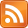SMS scnews item created by Daniel Daners at Fri 23 Mar 2018 1614
Type: Seminar
Distribution: World
Expiry: 27 Mar 2018
Calendar1: 27 Mar 2018
CalLoc1: AGR Carslaw 829
CalTitle1: PDE Seminar: Sharp bounds for Neumann eigenvalues (Brandolini)
Auth: daners@dora.maths.usyd.edu.au

# Sharp bounds for Neumann eigenvalues

### Brandolini

Barbara Brandolini
Università degli Studi di Napoli “Federico II”, Italy
Tue 27th Mar 2018, 2-3pm, Carslaw Room 829 (AGR)

## Abstract

We prove a sharp lower bound for the first nontrivial Neumann eigenvalue ${\mu }_{1}\left(\Omega \right)$ of the $p$-Laplace operator ($p>1$) in a Lipschitz, bounded domain $\Omega$ in ${ℝ}^{n}$. Differently from the pioneering estimate by Payne-Weinberger, our lower bound does not require any convexity assumption on $\Omega$, it involves the best isoperimetric constant relative to $\Omega$ and it is sharp, at least when $p=n=2$, as the isoperimetric constant relative to $\Omega$ goes to 0. Moreover, in a suitable class of convex planar domains, our estimate turns out to be better than the one provided by the Payne-Weinberger inequality.

Furthermore, we prove that, when $p=n=2$ and $\Omega$ consists of the points on one side of a smooth curve $\gamma$, within a suitable distance $\delta$ from it, then ${\mu }_{1}\left(\Omega \right)$ can be sharply estimated from below in terms of the length of $\gamma$, the ${L}^{\infty }$ norm of its curvature and $\delta$.

For Seminar announcements you can now subscribe to theSeminar RSS feed. Check also the PDE Seminar page.

Enquiries to Daniel Hauer or Daniel Daners.

If you are registered you may mark the scnews item as read.
School members may try to .